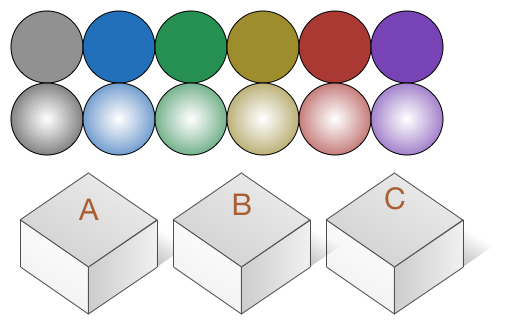# JEE-Mains 2015 (30/30) - Modified

Probability Level 2If 12 distinct balls are to be placed in 3 distinct boxes $A, B, C$, then the probability that box $A$ contains exactly 3 balls is?

×